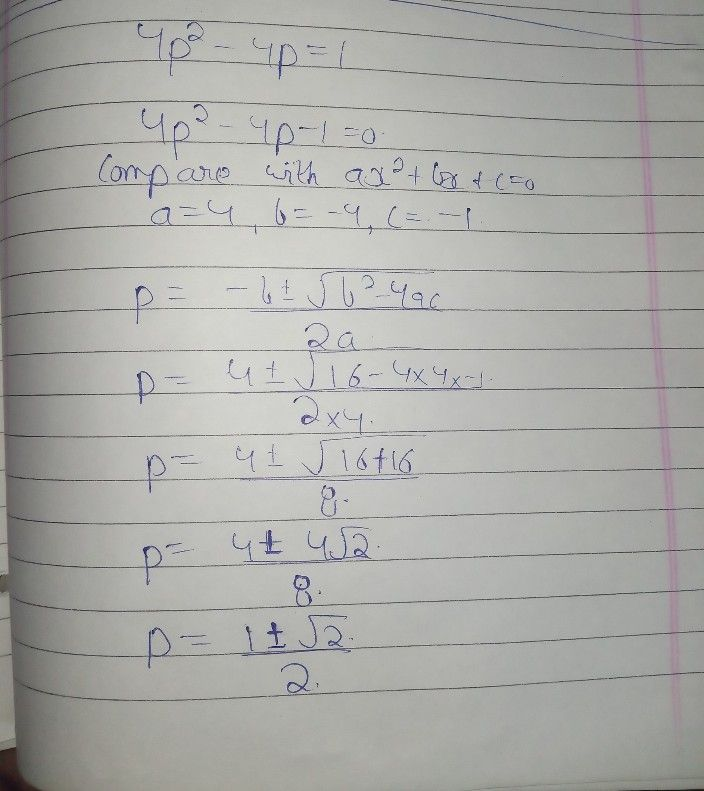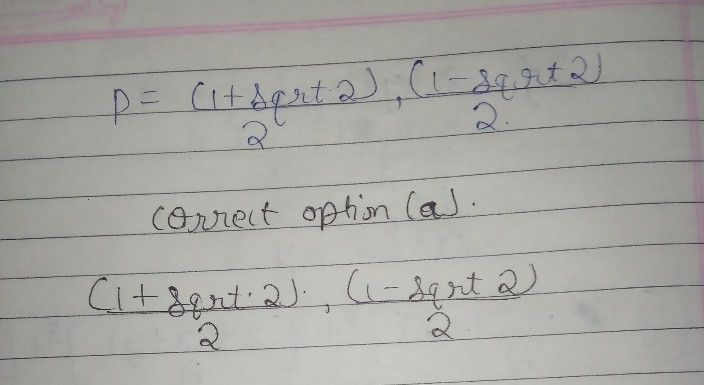Symbol
ProblemSolve. $4p^{2}-4p=1$ $O\left(1+sqr2\right)/2,\left(1.sqrt2\right)/2$ $O$ $\left(4+-sqrt32\right)/8$ $O$ $\left(4+.4sqr2\right)/8$ $0$ Prime
Algebra
Question content
Can you help me solve this, i’m confused and all the answers i’m getting aren’t matching the answer choices
SolutionQanda teacher - TrishaIf you have any doubts please do let me know dear. if you are satisfied with my answer please do LIKE and give a 5 STAR RATING and coins as THANK U GIFT..! ALL THE BEST..!!!
plz send coins as a giftStudent
thank you so much